MORE IN Basic Civil Engg. & Engg. Mechanics
RGPV First Year Engineering (Set B) (Semester 1)
Basic Civil Engg. & Engg. Mechanics
December 2011
Total marks: --
Total time: --
INSTRUCTIONS
(1) Assume appropriate data and state your reasons
(2) Marks are given to the right of every question
(3) Draw neat diagrams wherever necessary

Answer any one question from Q1 & Q2
1 (a) Mention and describe the various steps involved in production of concrete.
7 M
1 (b) Design a staircase for G+2 storey residential building. The clear height of ground floor and two upper floor is 3?30 m and 3?10 m respectively. Also draw longitudinal section of the designed staircase.
7 M

2 (a) Describe about the various field tests performed to access the quality of bricks. Also discuss about the classification of the bricks.
7 M
2 (b) What is the importance of foundation in a building? With the help of neat sketches explain about the constructional features of the under-reamed pile foundation.
7 M

Answer any one question from Q3 & Q4
3 (a) The following successive readings were taken with a dumpy level along a chain line at common intervals of 20 m. The R. L. of first station was 120-50 m. The dumpy level was shifted after third, sixth and eighth reading. Calculate R. L. of each station and also find gradient of the joining first and last station. The readings are:
0.945 m, 1.270 m, 1.890 m, 0.655 m, 1.355 m, 1.980 m, 0.730 m, 1.675 m, 0.985 m, 1.805 m, 2.150 m, 3.010 m.
7 M
3 (b) Discuss about the merits and demerits of plane table surveying. Also enumerate various accessories used in plane table surveying.
7 M

4 (a) Prove mathematically that the errors due to curvature and refractions are removed by reciprocal levelling. Derive suitable formula for finding true difference of elevation between two points using reciprocal levelling.
7 M
4 (b) Compare prismatic and surveyor's compass.
7 M

Answer any one question from Q5 & Q6
5 (a) Define and describe remote sensing. Explain its applications in various fields.
7 M
5 (b) What do you mean by contours? Describe about the characteristics of the contours.
7 M

6 (a) A series of offsets were taken from a chain line to a curved boundary line at intervals of 15 m in the following order:
0.00, 2.65, 3.80, 4.65, 3.60, 4.95, 5.85 metre compute the area between the chain line and the curved boundary and the end offset by:
i) Simpson's rule
ii) Trapezoidal rule
7 M
6 (b) Explain the following terms:
Profile levelling, Check lines, Tie line, GTS Benchmark, Well conditioned triangle, Local attraction error.
7 M

Answer any one question from Q7 & Q8
7 (a) Analyse the truss shown below. All members are of 30.0 m length.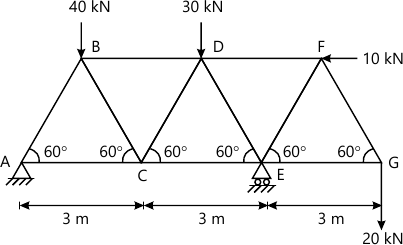7 M
7 (b) A 3000 N block is placed on an inclined plane as shown in figure. Find the maximum value of W for equilibrium if tipping does not occur. Assume coefficient of friction as 0.2.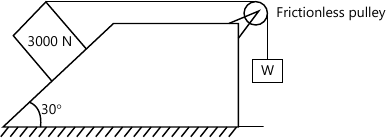7 M

8 (a) What do you mean by perfect, deficient and redundant frames? What are the assumption made in analysis of perfect frames?
7 M
8 (b) A beam AB supports a U.D.L. of intensity W1 and rests on soil which exerts a uniformly varying upward reaction as shown in figure. Determine W2 and W3 corresponding to equilibrium. Note that soil can exert only an upward reaction at any point on the beam. Hence state for what range of values of a/L the results obtained are valid.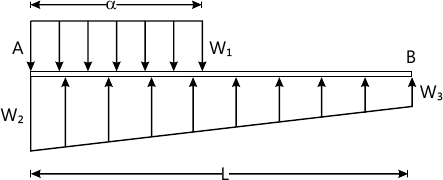7 M

Answer any one question from Q9 & Q10
9 (a) Draw the SF and BM diagram for beam shown in below mentioned figure. Find out the position and magnitude of maximum moment.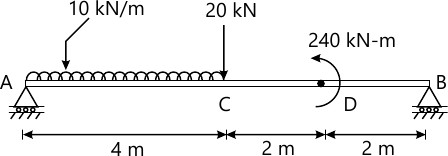7 M
9 (b) Find out the moment of inertia of circular lamina about its diametral axis using first principle.
7 M

10 (a) Determine the centroid of the section of the concrete dam shown in figure.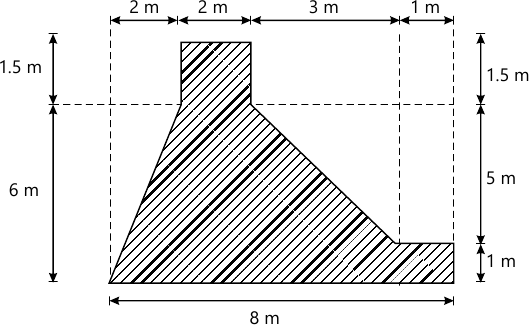7 M
10 (b) determine moment of inertia of the Z-section about the centroid axis X-X and Y-Y. All dimensions are in cm.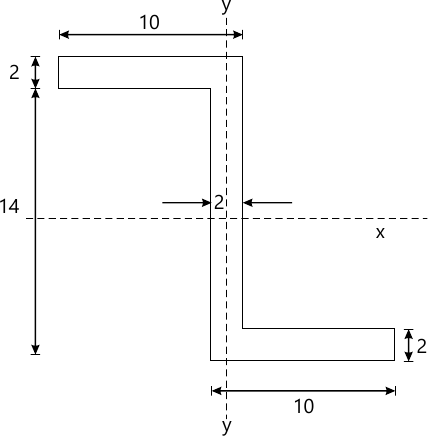7 M

More question papers from Basic Civil Engg. & Engg. Mechanics